﻿

### (DF)-Spaces of type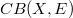$CB(X, E)$ and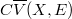$C\overline{V}(X, E)$

#### Abstract

Some locally convex properties of the spaces$CB( X, E)$ of the bounded continuous functions on a completely regular Hausdorff space X with values in a (DF-space) E are studied and applied to the (DF)-spaces of type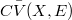$C\bar{V}(X,E)$ (e.g., see [S]).The following are our main results: 1.$CB(X,E)$ is a (DF)-space if and only if E is a (DF)-space. 2.For a (DF)-space E,$CB(X,E)$ is quasi barrelled if and only if either (i)X is pseudocompact and E is quasibarrelled or (ii) X is not pseudocompact and the bounded subsets of E are metrizaable. 3. If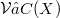$\mathcal V ⊂ C(X)$ and if each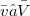$\bar{v}∈\bar{V}$ is dominated by some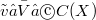$\tilde{v}∈ \bar{V}∩ C(X)$, then$C\bar{V}(X,E)$ (resp.,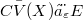$C\bar{V}(X)⨂_\varepsilon E$) is a (DF)-space if and only if E is a (DF)-space. 4. Let X be a locally compact and σ-compact space,$\mathcal V ⊂ C(X)$ and E a (DF)-space. Then$C\bar{V}(X,E)$ is quasibarrelled if and only if (i) E is quasibarrelled and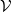$\mathcal V$ satisfies condition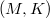$( M, K)$ or (ii) the bounded subsets of E are metrizable and$\mathcal V$ satisfies condition (D).

DOI Code: 10.1285/i15900932v10supn1p127

Full Text: PDF